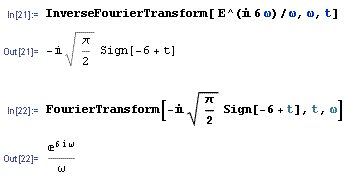# Inverse Fourier transform

Telemachus
Hi there. I have some trouble with this. I have to find the inverse Fourier transform for: $$\frac{e^{i 6\omega}}{\omega}$$
So I'm using a table, then:
$$F^{-1}\left ( \frac{e^{i 6\omega}}{\omega}\right )=F^{-1}\left ( e^{i 6\omega}\right ) * F^{-1}\left ( \frac{1}{\omega}\right )=2\pi\left[ \delta (t+6)*\frac{i}{2}sg(t)\right]$$ where (*) represents the convolution.

Well, everything fine till there, but when I tried to corroborate my result with mathematica I get:I don't know what's wrong.

#### Attachments

Telemachus
I don't know which integral you are referring. I'm using that $$F(sg(t))=\frac{2}{i \omega}$$ and $$F(\delta(t-t_0))=e^{-i \omega t_0}$$

Then $$F^{-1}(e^{i6\omega})=\delta(t+6)$$ and $$F^{-1}\left (\frac{1}{\omega}\right )=F^{-1}\left ( \frac{i}{2}\frac{2}{i \omega}\right )=\frac{i}{2}sg(t)$$

Homework Helper
The convolution is given by:
$$f\star g(x)=\int_{-\infty}^{\infty}f(y)(g(x-y)dy$$
Then the Fourier transform is just the product of the Fourier transforms of f and g. So in this case you have $\hat{f}(\omega )=e^{6\omega i}$ and $\hat{g}(\omega )=\omega^{-1}$ and so the founction you're tring to find is:
$$i\sqrt{\frac{\pi}{2}}\int_{\infty}^{\infty}\delta (y+6)\textrm{sign}(y-x)dy$$

Telemachus
$$f\star g(x)=\int_{-\infty}^{\infty}f(y)(g(x-y)dy$$

But I don't know how you get this from there:

$$i\sqrt{\frac{\pi}{2}}\int_{\infty}^{\infty}\delta (y+6)\textrm{sign}(y-x)dy$$
Particularly this: $$i\sqrt{\frac{\pi}{2}}$$
I don't know where you get that from.

I get from here: $$2\pi\left[ \delta (t+6)*\frac{i}{2}sg(t)\right]=i \pi \int_{\infty}^{\infty}\delta (t+6)\textrm{sign}(x-t)dy$$ so I make $$x_0=-6$$, then:
$$i \pi \int_{\infty}^{\infty}\delta (x+6)\textrm{sign}(t-x)dx=i \pi \textrm{Sign}(t+6)$$

Last edited:
MisterX
Edit: incorrect

Last edited:
Telemachus
I think it's fine. Look, I have the table right here.

$$F^{-1}(e^{-i\omega t_0})=\delta(t-t_0)$$
Then I have $$t_0=-6$$

Thats what I've used.

MisterX
Yes, I think I made a mistake. I don't know how Mathematica arrives at that result.

Telemachus
Yes, I don't know neither. And actually the problem is I don't know where I have the mistake, I think that my solution is fine, maybe I'm not doing it well in mathematica, I'm thinking that there are different ways for defining a Fourier transform. You can use the frequencies or the angular frequency. Maybe I'm just not doing it well with mathematica, perhaps I have to define something else, that's a possibility.

Homework Helper
Dearly Missed
Hi there. I have some trouble with this. I have to find the inverse Fourier transform for: $$\frac{e^{i 6\omega}}{\omega}$$
So I'm using a table, then:
$$F^{-1}\left ( \frac{e^{i 6\omega}}{\omega}\right )=F^{-1}\left ( e^{i 6\omega}\right ) * F^{-1}\left ( \frac{1}{\omega}\right )=2\pi\left[ \delta (t+6)*\frac{i}{2}sg(t)\right]$$ where (*) represents the convolution.

Well, everything fine till there, but when I tried to corroborate my result with mathematica I get:I don't know what's wrong.

Here is what I get using Maple:

fw:=exp(I*6*w)/w;
exp(6 I w)
fw := ----------
w

> ft:=invfourier(fw,w,t);

ft := 1/2 I (2 Heaviside(6 + t) - 1)

> convert(%,piecewise);

{ -1/2 I t < -6
{
{ undefined I t = -6
{
{ 1/2 I -6 < t

RGV

Telemachus
Thats even weirder.

Staff Emeritus
Homework Helper
If you're just wondering about the constants out front, you have to remember there are various conventions of where to put the $2\pi$. Mathematica has a parameter called, appropriately enough, FourierParameters that you can set depending on which convention you want to use.

Telemachus
Thank you vela :) do you have any idea about how mathematica gets to that? I think I should read more carefully the help content on this topic, but I don't have to much time for this, so maybe if you know the underlying formalism for this I'll appreciate if you let me know :P

Does it have any sense the solution with the heaviside function that Ray found using Maple? that's not a constant difference, but I'm aware of the similarities between the unit step function and the sign function, they differ only for the negative real values (I have no idea about what happens on the complex field).

Last edited:
Staff Emeritus
Homework Helper
By default, this is what Mathematica uses for the inverse Fourier transform:

$$f(t) = \frac{1}{\sqrt{2\pi}}\int_{-\infty}^\infty F(\omega)e^{-i\omega t}\,d\omega$$
So Mathematica would give you, for example,

$$F^{-1}[e^{i6\omega}] = \frac{1}{\sqrt{2\pi}}\int_{-\infty}^\infty e^{i6\omega}e^{-i\omega t}\,d\omega = \sqrt{2\pi}\left[\frac{1}{2\pi}\int_{-\infty}^\infty e^{i(6-t)\omega}\,d\omega\right]$$
where the quantity in the square brackets is the Dirac delta function $\delta(t-6)$.

From looking at the results you found in the table, I think you're using the convention

$$f(t) = \int_{-\infty}^\infty F(\omega)e^{i\omega t}\,d\omega$$
If you look in the Mathematica documentation on integral transforms, there's a list of what you want to set FourierParameters to to follow the convention you're using in your class.

As to the unit step and sign functions, they're related by a vertical shift and vertical scaling. You should be able to figure that out pretty easily.

Homework Helper
The definition of the integral transform I am using is:
$$\hat{f}(\omega )=\int_{-\infty}^{\infty}f(x)e^{-i\omega x}dx$$
All I did was take the information from the wikipedia page and use that. The coefficient $i\sqrt{\pi /2}$ came from examining the Fourier transform of the sign function.

Telemachus
Thank you vela.
hunt_mat, I think wikipedia uses the formalism that vela just mentioned. You used the Fourier transform for the sign that you found in wikipedia or the one I've posted?

Homework Helper
having said that now, I am a little unsure of the sign I used.